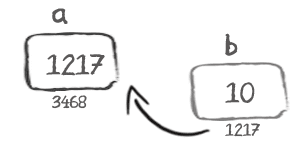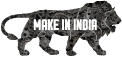C Pointers Lesson
Sunday, September 07, 2008

# C Pointers Lesson

Pointer is a special variable in which the value stored are the addresses of some other variable(or function also in some cases)
int *a;

The statement written above means that a is a special variable(i.e. pointer) that will store an address of another variable which is integer.
For eg.

int *a;
int b=10;
a=&b;

then it will be like this-Here 3465 is address for variable a and 1217 is address for variable b.

NOTE:All variables store integers bcoz address are always integers....
thus if we write

char *a;

It will not mean that a will store character it means that it will store the address of some variable whose datatype is char.

1.OK so far, but I think someone that this helped would need a lot more - for example the dreaded pointer arithmetic - dreaded because it causes so many bugs.

Eg why say
int * p;
compared with
char * p1;

whne they are both just pointers? Because
*(p+1) is the next int, and
*(p1+1) is the next char ie the declared type controls how pointer arithmetic works. A beginner woul dneed a lot more explanationX